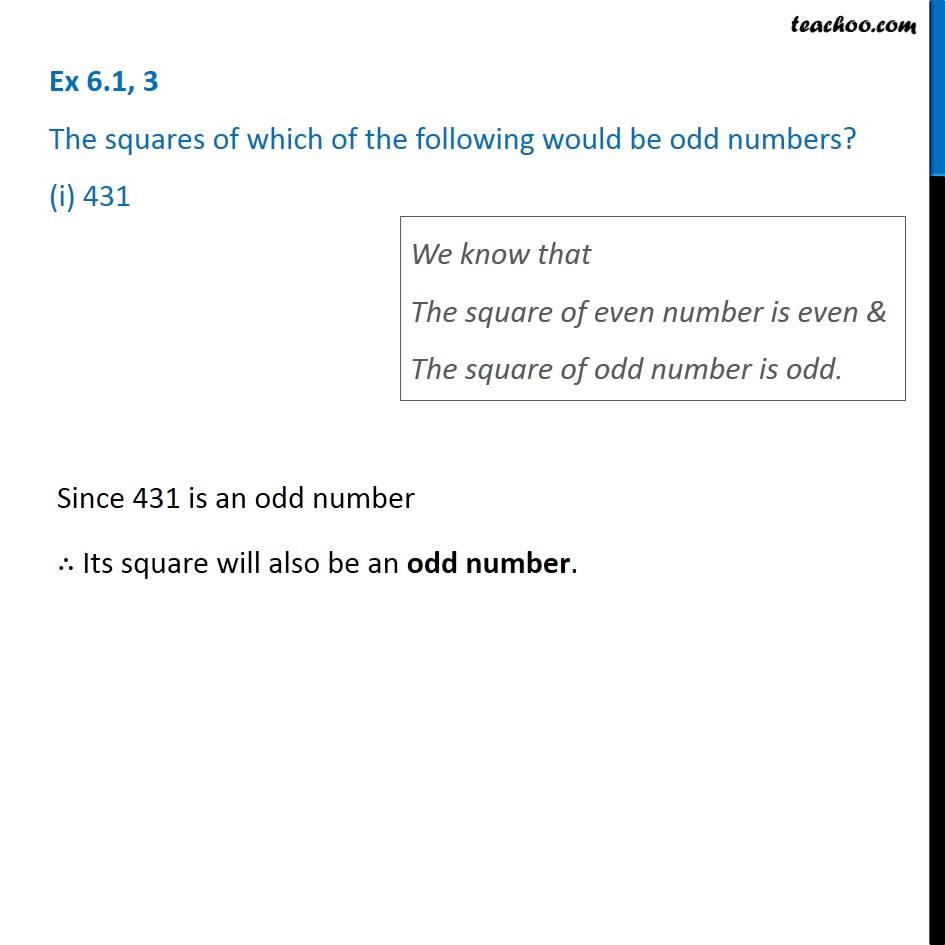1. Chapter 6 Class 8 Squares and Square Roots
2. Concept wise
3. Properties of square numbers

Transcript

Ex 6.1, 3 The squares of which of the following would be odd numbers? (i) 431 We know that The square of even number is even & The square of odd number is odd. Since 431 is an odd number ∴ Its square will also be an odd number. Since 431 is an odd number ∴ Its square will also be an odd number.